### Notation for the Item Response Theory Model

This section introduces the mathematical notation that is used throughout the chapter to describe the item response theory (IRT) model. For a description of the fitting algorithms and the mathematical-statistical details, see the section Details: IRT Procedure.

A d-dimensional IRT model that has J ordinal responses can be expressed by the equations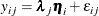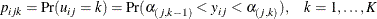where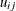is the observed ordinal response from subject i for item j,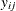is a continuous latent response that underlies,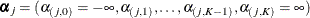is a vector of threshold parameters for item j,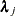is a vector of slope (or discrimination) parameters for item j,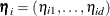is a vector of latent factors for subject i,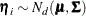, and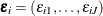is a vector of unique factors for subject i. All the unique factors in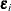are independent from one another, suggesting that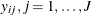, are independent conditional on the latent factor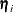. This is the so-called local independence assumption. Finally,andare also independent.

Based on the preceding model specification,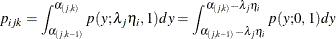where
is determined by the link function. It is the density function of the standard normal distribution if the probit link is used, or the density function of the logistic distribution if the logistic link is used.

Let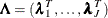denote the slope matrix. To identify the model in exploratory analysis, the upper triangular elements of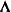are fixed as zero, the factor means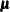is fixed as a zero vector, and the factor variance covariance matrix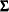is fixed as an identity matrix. For confirmatory analysis, it is assumed that the identification problem is solved by user-specified constraints.

The model that is specified in the preceding equation is called the multidimensional graded response model. When the responses are binary and there is only one latent factor, this model reduces to the two-parameter model, which can be expressed as follows: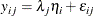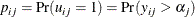A different parameterization for the two-parameter model is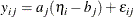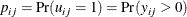where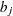is interpreted as item difficulty and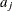is called the discrimination parameter. The preceding two parameterizations are mathematically equivalent. For binary response items, you can transfer the threshold parameter into the difficulty parameter by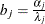. The IRT procedure uses the first parameterization.

The two-parameter model reduces to a one-parameter model when slope parameters for all the items are constrained to be equal. In the case where logistic link is used, the one- and two-parameter models are often abbreviated as 1PL and 2PL. When all the slope parameters are set to 1 and the factor variance is set to a free parameter, the Rasch model is obtained.

You can obtain three- and four-parameter models by introducing the guessing and ceiling parameters. Let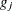and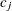denote the item-specific guessing and ceiling parameters. Then the four-parameter model can be expressed as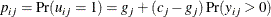This model reduces to the three-parameter model when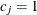.